CHAPTER 1. Mathematics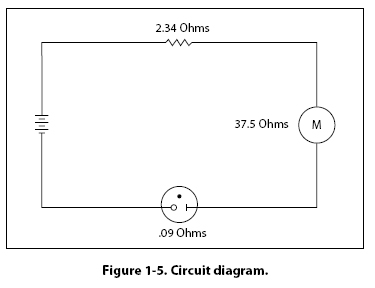Addition of Decimal Numbers To add decimal numbers, they must first be arranged so that the decimal points are aligned vertically and according to place value. That is, adding tenths with tenths, ones with ones, hundreds with hundreds, and so forth. Example: Find the total resistance for the circuit diagram shown in Figure 1-5. The total resistance of a series circuit is equal to the sum of the individual resistances. To find the total resistance, RT, the individual resistances are added together.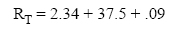Arrange the resistance values in a vertical column so that the decimal points are aligned and then add.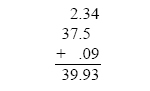Therefore, the total resistance, RT = 39.93 ohms. Subtraction of Decimal Numbers To subtract decimal numbers, they must first be arranged so that the decimal points are aligned vertically and according to place value. That is, subtracting tenths from tenths, ones from ones, hundreds from hundreds, and so forth. Example: A series circuit containing two resistors has a total resistance (RT) of 37.272 ohms. One of the resistors (R1) has a value of 14.88 ohms. What is the value of the other resistor (R2)?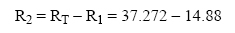Arrange the decimal numbers in a vertical column so that the decimal points are aligned and then subtract.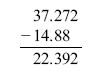Therefore, the second resistor, R2 = 22.392 ohms. Multiplication of Decimal Numbers To multiply decimal numbers, vertical alignment of the decimal point is not required. Instead, align the numbers to the right in the same way as whole numbers are multiplied (with no regard to the decimal points or place values) and then multiply. The last step is to place the decimal point in the correct place in the answer. To do this, “count" the number of decimal places in each of the numbers, add the total, and then “give" that number of decimal places to the result. Example: To multiply 0.2 x 6.03, arrange the numbers vertically and align them to the right. Multiply the numbers, ignoring the decimal points for now.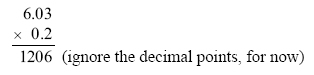After multiplying the numbers, count the total number of decimal places in both numbers. For this example, 6.03 has 2 decimal places and 0.2 has 1 decimal place. Together there are a total of 3 decimal places. The decimal point for the answer will be placed 3 decimal places from the right. Therefore, the answer is 1.206.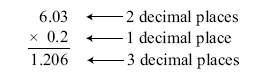Example: Using the formula Watts = Amperes x Voltage, what is the wattage of an electric drill that uses 9.45 amperes from a 120 volt source? Align the numbers to the right and multiply. After multiplying the numbers, count the total number of decimal places in both numbers. For this example, 9.45 has 2 decimal places and 120 has no decimal place. Together there are 2 decimal places. The decimal point for the answer will be placed 2 decimal places from the right. Therefore, the answer is 1,134.00 watts, or 1,134 watts.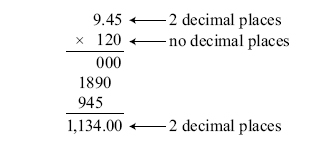©AvStop Online Magazine                                                                                                                                                      Contact Us              Return To Books